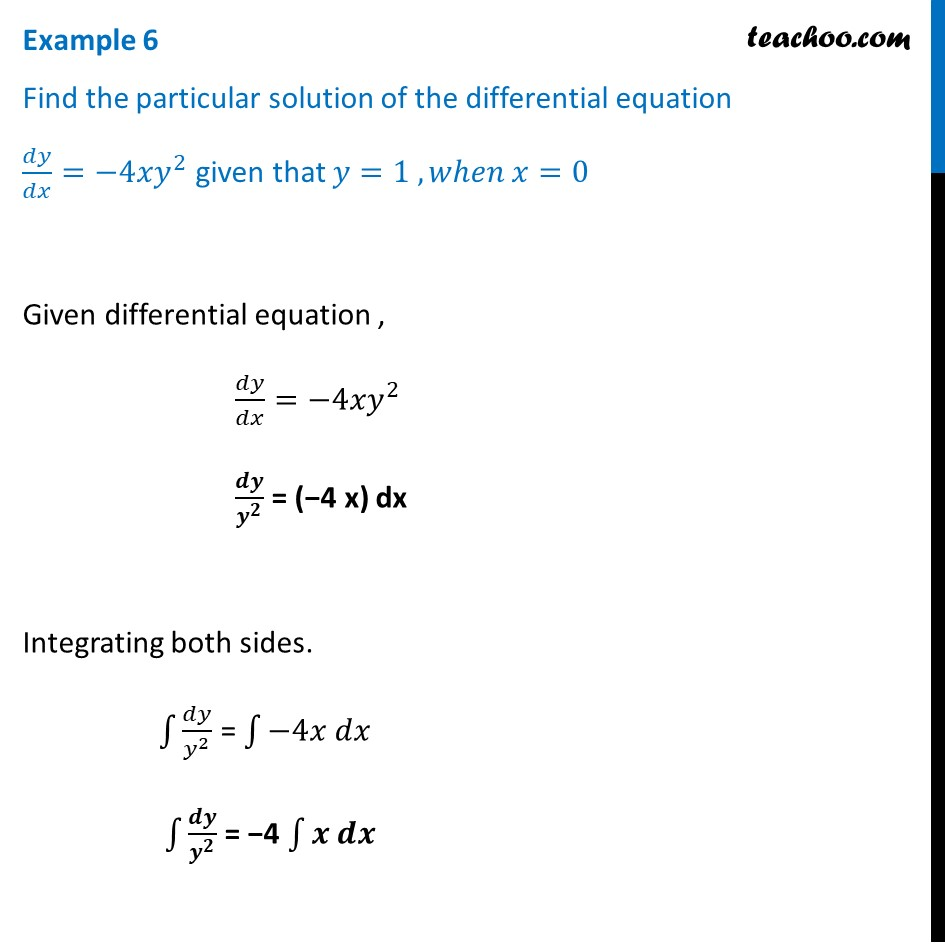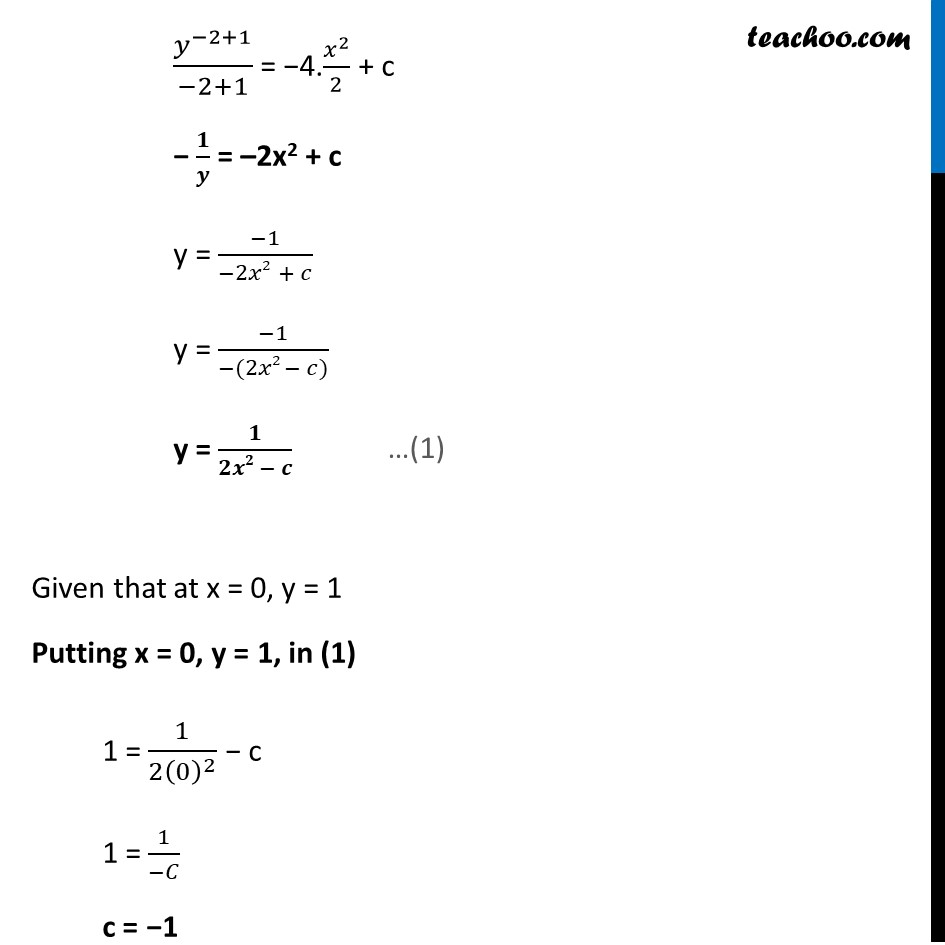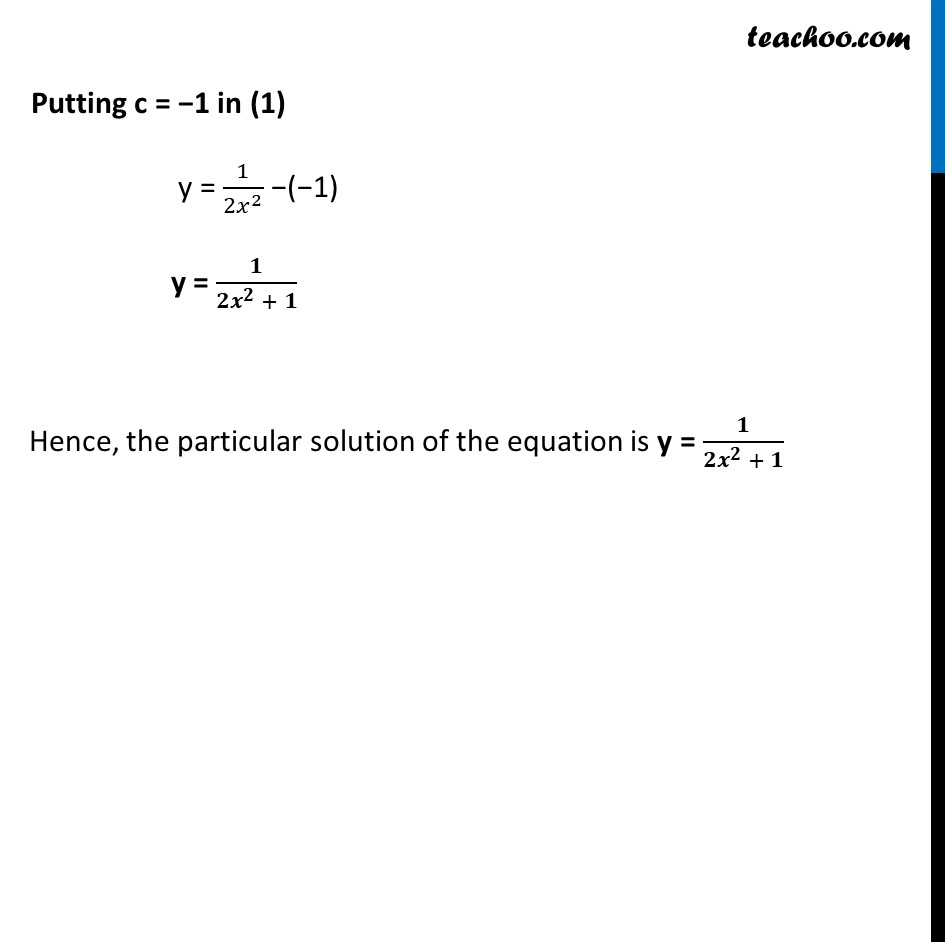Examples

Chapter 9 Class 12 Differential Equations
Serial order wiseLearn in your speed, with individual attention - Teachoo Maths 1-on-1 Class

### Transcript

Example 6 Find the particular solution of the differential equation 𝑑𝑦/𝑑𝑥=−4𝑥𝑦^2 given that 𝑦=1 , 𝑤ℎ𝑒𝑛 𝑥=0Given differential equation , 𝑑𝑦/𝑑𝑥=−4𝑥𝑦^2 𝒅𝒚/𝒚^𝟐 = (−4 x) dx Integrating both sides. ∫1▒𝑑𝑦/𝑦^2 = ∫1▒〖−4𝑥 𝑑𝑥〗 ∫1▒𝒅𝒚/𝒚^𝟐 = −4 ∫1▒〖𝒙 𝒅𝒙〗 𝑦^(−2+1)/(−2+1) = −4.𝑥^2/2 + c − 𝟏/𝒚 = –2x2 + c y = (−1)/(−2𝑥2 + 𝑐) y = (−1)/(−(2𝑥2 − 𝑐)) y = 𝟏/(𝟐𝒙𝟐 − 𝒄) Given that at x = 0, y = 1 Putting x = 0, y = 1, in (1) 1 = 1/(2(0)^2 ) − c 1 = 1/(−𝐶) c = −1 Putting c = −1 in (1) y = 1/(2𝑥^2 ) −(−1) y = 𝟏/(𝟐𝒙^𝟐 + 𝟏) Hence, the particular solution of the equation is y = 𝟏/(𝟐𝒙^𝟐 + 𝟏)